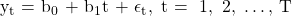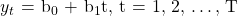Essential Concept 6: Linear vs Log-Linear Trend Models | IFT World
101 Concepts for the Level I Exam

# Essential Concept 6: Linear vs Log-Linear Trend Models• When the dependent variable changes at a constant amount with time, a linear trend model is used.
The linear trend equation is given by• When the dependent variable changes at a constant rate (grows exponentially), a log-linear trend model is used.
The log-liner trend equation is given by ln• A limitation of trend models is that by nature they tend to exhibit serial correlation in errors, due to which they are not useful.
• The Durban-Watson statistic is used to test for serial correlation. If this statistic differs significantly from 2, then we can conclude the presence of serial correlation in errors. To overcome this problem, we use autoregressive time series (AR) models.

Crash Courses for November CFA Level I, II exams are coming soon!
This is default text for notification bar